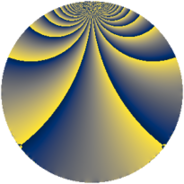# Properties

 Label 966.2.q.iLevel $966$ Weight $2$ Character orbit 966.q Analytic conductor $7.714$ Analytic rank $0$ Dimension $40$ CM no Inner twists $2$

# Related objects

## Newspace parameters

 Level: $$N$$ $$=$$ $$966 = 2 \cdot 3 \cdot 7 \cdot 23$$ Weight: $$k$$ $$=$$ $$2$$ Character orbit: $$[\chi]$$ $$=$$ 966.q (of order $$11$$, degree $$10$$, minimal)

## Newform invariants

 Self dual: no Analytic conductor: $$7.71354883526$$ Analytic rank: $$0$$ Dimension: $$40$$ Relative dimension: $$4$$ over $$\Q(\zeta_{11})$$ Twist minimal: yes Sato-Tate group: $\mathrm{SU}(2)[C_{11}]$

## $q$-expansion

The dimension is sufficiently large that we do not compute an algebraic $$q$$-expansion, but we have computed the trace expansion.

 $$\operatorname{Tr}(f)(q) =$$ $$40q - 4q^{2} - 4q^{3} - 4q^{4} + 4q^{5} - 4q^{6} - 4q^{7} - 4q^{8} - 4q^{9} + O(q^{10})$$ $$\operatorname{Tr}(f)(q) =$$ $$40q - 4q^{2} - 4q^{3} - 4q^{4} + 4q^{5} - 4q^{6} - 4q^{7} - 4q^{8} - 4q^{9} + 4q^{10} - q^{11} - 4q^{12} - 8q^{13} - 4q^{14} - 7q^{15} - 4q^{16} - 7q^{17} - 4q^{18} + 20q^{19} - 7q^{20} - 4q^{21} + 10q^{22} + 2q^{23} + 40q^{24} - 22q^{25} + 14q^{26} - 4q^{27} - 4q^{28} + 15q^{29} + 4q^{30} - 16q^{31} - 4q^{32} - q^{33} - 7q^{34} - 7q^{35} - 4q^{36} - 16q^{37} - 13q^{38} - 8q^{39} + 4q^{40} - 17q^{41} - 4q^{42} + 26q^{43} - q^{44} + 4q^{45} - 20q^{46} + 72q^{47} - 4q^{48} - 4q^{49} + 11q^{50} - 7q^{51} - 19q^{52} + 6q^{53} - 4q^{54} + 49q^{55} - 4q^{56} + 9q^{57} - 7q^{58} - 51q^{59} + 4q^{60} - 42q^{61} - 16q^{62} - 4q^{63} - 4q^{64} + 8q^{65} - 12q^{66} + 54q^{67} + 4q^{68} + 2q^{69} + 4q^{70} - 59q^{71} - 4q^{72} - 27q^{73} - 16q^{74} + 11q^{75} - 2q^{76} - 12q^{77} - 8q^{78} - 6q^{79} - 7q^{80} - 4q^{81} - 6q^{82} - 24q^{83} - 4q^{84} + 35q^{85} - 7q^{86} - 29q^{87} - q^{88} + 22q^{89} - 7q^{90} + 36q^{91} - 9q^{92} + 50q^{93} - 16q^{94} + 22q^{95} - 4q^{96} + 16q^{97} - 4q^{98} - q^{99} + O(q^{100})$$

## Embeddings

For each embedding $$\iota_m$$ of the coefficient field, the values $$\iota_m(a_n)$$ are shown below.

For more information on an embedded modular form you can click on its label.

Label $$a_{2}$$ $$a_{3}$$ $$a_{4}$$ $$a_{5}$$ $$a_{6}$$ $$a_{7}$$ $$a_{8}$$ $$a_{9}$$ $$a_{10}$$
85.1 −0.654861 + 0.755750i 0.841254 0.540641i −0.142315 0.989821i −1.70670 3.73716i −0.142315 + 0.989821i −0.959493 + 0.281733i 0.841254 + 0.540641i 0.415415 0.909632i 3.94201 + 1.15748i
85.2 −0.654861 + 0.755750i 0.841254 0.540641i −0.142315 0.989821i −0.446804 0.978364i −0.142315 + 0.989821i −0.959493 + 0.281733i 0.841254 + 0.540641i 0.415415 0.909632i 1.03199 + 0.303020i
85.3 −0.654861 + 0.755750i 0.841254 0.540641i −0.142315 0.989821i 0.431857 + 0.945634i −0.142315 + 0.989821i −0.959493 + 0.281733i 0.841254 + 0.540641i 0.415415 0.909632i −0.997469 0.292883i
85.4 −0.654861 + 0.755750i 0.841254 0.540641i −0.142315 0.989821i 1.56679 + 3.43079i −0.142315 + 0.989821i −0.959493 + 0.281733i 0.841254 + 0.540641i 0.415415 0.909632i −3.61885 1.06259i
127.1 0.841254 0.540641i −0.142315 + 0.989821i 0.415415 0.909632i −2.99676 + 0.879928i 0.415415 + 0.909632i −0.654861 0.755750i −0.142315 0.989821i −0.959493 0.281733i −2.04531 + 2.36041i
127.2 0.841254 0.540641i −0.142315 + 0.989821i 0.415415 0.909632i −1.32090 + 0.387851i 0.415415 + 0.909632i −0.654861 0.755750i −0.142315 0.989821i −0.959493 0.281733i −0.901523 + 1.04041i
127.3 0.841254 0.540641i −0.142315 + 0.989821i 0.415415 0.909632i 2.16398 0.635401i 0.415415 + 0.909632i −0.654861 0.755750i −0.142315 0.989821i −0.959493 0.281733i 1.47693 1.70447i
127.4 0.841254 0.540641i −0.142315 + 0.989821i 0.415415 0.909632i 3.49494 1.02621i 0.415415 + 0.909632i −0.654861 0.755750i −0.142315 0.989821i −0.959493 0.281733i 2.38532 2.75280i
169.1 −0.142315 + 0.989821i 0.415415 + 0.909632i −0.959493 0.281733i −1.80470 2.08274i −0.959493 + 0.281733i 0.841254 + 0.540641i 0.415415 0.909632i −0.654861 + 0.755750i 2.31838 1.48993i
169.2 −0.142315 + 0.989821i 0.415415 + 0.909632i −0.959493 0.281733i −0.987362 1.13948i −0.959493 + 0.281733i 0.841254 + 0.540641i 0.415415 0.909632i −0.654861 + 0.755750i 1.26839 0.815148i
169.3 −0.142315 + 0.989821i 0.415415 + 0.909632i −0.959493 0.281733i 0.751750 + 0.867566i −0.959493 + 0.281733i 0.841254 + 0.540641i 0.415415 0.909632i −0.654861 + 0.755750i −0.965721 + 0.620631i
169.4 −0.142315 + 0.989821i 0.415415 + 0.909632i −0.959493 0.281733i 2.39800 + 2.76744i −0.959493 + 0.281733i 0.841254 + 0.540641i 0.415415 0.909632i −0.654861 + 0.755750i −3.08054 + 1.97974i
211.1 0.415415 + 0.909632i −0.959493 + 0.281733i −0.654861 + 0.755750i −2.63117 1.69095i −0.654861 0.755750i −0.142315 0.989821i −0.959493 0.281733i 0.841254 0.540641i 0.445115 3.09584i
211.2 0.415415 + 0.909632i −0.959493 + 0.281733i −0.654861 + 0.755750i −0.627520 0.403283i −0.654861 0.755750i −0.142315 0.989821i −0.959493 0.281733i 0.841254 0.540641i 0.106158 0.738342i
211.3 0.415415 + 0.909632i −0.959493 + 0.281733i −0.654861 + 0.755750i 1.41748 + 0.910960i −0.654861 0.755750i −0.142315 0.989821i −0.959493 0.281733i 0.841254 0.540641i −0.239795 + 1.66781i
211.4 0.415415 + 0.909632i −0.959493 + 0.281733i −0.654861 + 0.755750i 2.75663 + 1.77158i −0.654861 0.755750i −0.142315 0.989821i −0.959493 0.281733i 0.841254 0.540641i −0.466338 + 3.24345i
463.1 −0.142315 0.989821i 0.415415 0.909632i −0.959493 + 0.281733i −1.80470 + 2.08274i −0.959493 0.281733i 0.841254 0.540641i 0.415415 + 0.909632i −0.654861 0.755750i 2.31838 + 1.48993i
463.2 −0.142315 0.989821i 0.415415 0.909632i −0.959493 + 0.281733i −0.987362 + 1.13948i −0.959493 0.281733i 0.841254 0.540641i 0.415415 + 0.909632i −0.654861 0.755750i 1.26839 + 0.815148i
463.3 −0.142315 0.989821i 0.415415 0.909632i −0.959493 + 0.281733i 0.751750 0.867566i −0.959493 0.281733i 0.841254 0.540641i 0.415415 + 0.909632i −0.654861 0.755750i −0.965721 0.620631i
463.4 −0.142315 0.989821i 0.415415 0.909632i −0.959493 + 0.281733i 2.39800 2.76744i −0.959493 0.281733i 0.841254 0.540641i 0.415415 + 0.909632i −0.654861 0.755750i −3.08054 1.97974i
See all 40 embeddings
 $$n$$: e.g. 2-40 or 990-1000 Embeddings: e.g. 1-3 or 883.4 Significant digits: Format: Complex embeddings Normalized embeddings Satake parameters Satake angles

## Inner twists

Char Parity Ord Mult Type
1.a even 1 1 trivial
23.c even 11 1 inner

## Twists

By twisting character orbit
Char Parity Ord Mult Type Twist Min Dim
1.a even 1 1 trivial 966.2.q.i 40
23.c even 11 1 inner 966.2.q.i 40

By twisted newform orbit
Twist Min Dim Char Parity Ord Mult Type
966.2.q.i 40 1.a even 1 1 trivial
966.2.q.i 40 23.c even 11 1 inner

## Hecke kernels

This newform subspace can be constructed as the kernel of the linear operator $$15\!\cdots\!35$$$$T_{5}^{15} + 864283892383 T_{5}^{14} -$$$$29\!\cdots\!54$$$$T_{5}^{13} + 540929649503 T_{5}^{12} +$$$$10\!\cdots\!24$$$$T_{5}^{11} +$$$$93\!\cdots\!97$$$$T_{5}^{10} +$$$$69\!\cdots\!60$$$$T_{5}^{9} +$$$$26\!\cdots\!08$$$$T_{5}^{8} +$$$$26\!\cdots\!76$$$$T_{5}^{7} +$$$$45\!\cdots\!60$$$$T_{5}^{6} +$$$$24\!\cdots\!28$$$$T_{5}^{5} +$$$$41\!\cdots\!84$$$$T_{5}^{4} +$$$$35\!\cdots\!00$$$$T_{5}^{3} +$$$$23\!\cdots\!60$$$$T_{5}^{2} +$$$$90\!\cdots\!80$$$$T_{5} +$$$$68\!\cdots\!96$$">$$T_{5}^{40} - \cdots$$ acting on $$S_{2}^{\mathrm{new}}(966, [\chi])$$.